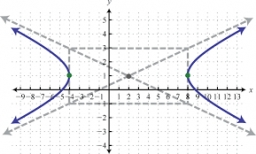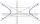# Equation - inverse

Solve for x:

7: x = 14: 1000

Correct result:

x =  500

#### Solution:

$7: x=14: 1000 \ \\ x \ne 0 \ \\ \ \\ \ \\ x/7=1000/14 \ \\ \ \\ 2x=1000 \ \\ \ \\ x=500$We would be pleased if you find an error in the word problem, spelling mistakes, or inaccuracies and send it to us. Thank you!Tips to related online calculators
Need help calculate sum, simplify or multiply fractions? Try our fraction calculator.
Do you have a linear equation or system of equations and looking for its solution? Or do you have quadratic equation?

#### You need to know the following knowledge to solve this word math problem:

We encourage you to watch this tutorial video on this math problem:

## Next similar math problems:

• HyperbolaFind the equation of hyperbola that passes through the point M [30; 24] and has focal points at F1 [0; 4 sqrt 6], F2 [0; -4 sqrt 6].
• Hyperbola equationFind the hyperbola equation with the center of S [0; 0], passing through the points: A [5; 3] B [8; -10]